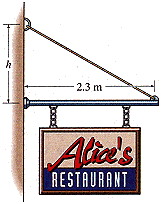# Need help equilibrium physics

jtulibarri
a 65 kg sign hung centered from a uniform rod of mass 9.8 kg and length 2.3 m. At one end(left) the rod is attached to the wall by a pivot; at the other end(right) it's supported by a cable that can withstand a maximum tension of 760 N. What is the minimum height h above the pivot for anchoring the cable to the wall?i need help on setting up an equation to solve...
what i have so far i don't know how wrong or right it is.

(637N)(1.15m)+(96.04N)(1.15m)-m*g*sin(angle away from wall)=0

? i don't really know exactly where I am going with this

Homework Helper
(637N)(1.15m)+(96.04N)(1.15m)-m*g*sin(angle away from wall)=0

? i don't really know exactly where I am going with this

you need the torque about that point to be zero... so you need:

(Force of sign)(Radius of sign)+(Force of rod)(Radius of rod)-(vertical component of Tension)*distance=0

(since horizontal component of tension exerts 0 torque about the pivot)

what is the vertical component of Tension in terms of the total tension T... what is the distance here?